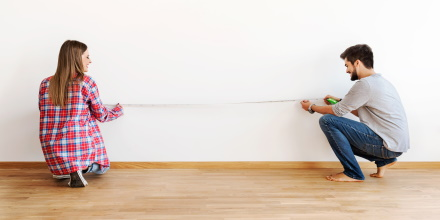# How much does it matter if the measuring tape sags?There are a couple ways in which a measurement might not be straight. Yesterday I wrote a blog post about not measuring straight toward your target. You’d like to measure from (0, 0) to (x, 0), but something is in the way, and so you measure from (0, 0) to (x, y), where y is small relative to x. We assumed the tape measure was straight, but didn’t aim straight at the target.

In this post we will consider measuring from (0, y) to (x, y), but with the tape measure sagging to (x/2, 0) in the middle. We end exactly at our target, but the tape bends. As before, we’ll assume y is small relative to x. About how large will our error be as a function of y?

My first thought was to assume our tape measure takes the shape of a catenary, because that’s the shape of a handing cable. But the resulting calculations are too complicated. The calculations depend on solving for a scaling factor, and that calculation cannot be done in closed form.

Since we are assuming the amount of sag y is small relative to the horizontal distance x, a parabola will do just as well as a catenary. (More on how well a parabola approximates a catenary here.)

The equation of the parabola passing through our three specified points, as a function of t, is

4y(tx/2)² / x².

Even with our simplifying assumption that the tape bends like a parabola, the arclength calculation is still a little tedious, but if I’ve done things correctly the result turns out to be

x + 32y² / 3x + higher order terms

So the error is on the order of (32/3) y²/x, where as in the earlier post the error was on the order of (1/2) y²/x. If y is small relative to x, the error is still small, but about 20x larger than before.

Going back to our example of measuring a 10 ft (120 inch) room, we want to measure from (0, 0) to (120, 0). If we measure with a straight line from (0, 0) to (120, 4) instead, there will be an error of about 1/15 of an inch. If instead we measure from (0, 4) to (120, 0) with a tape that sags like a parabola touching (60, 0), then the error will be closer to an inch and a half. But if we could pull tight enough to limit the sag to 1 inch, the measurement error would be more like 1/10 of an inch.

## Related posts

I inadvertently ended up writing three blog posts in a row related to measuring tapes. Here are the other two:

## 4 thoughts on “How much does it matter if the measuring tape sags?”

1. Alfredo Louro

Length dilation due to gravity. Isn’t that a relativistic effect?

2. Just remember that if you pull on the ends of the tape to try and make it perfectly straight the vector forces go toward infinity and the tape will break before it is perfectly staight so you must consider that in your calculations.

3. Dave Pawson

“The calculations depend on solving for a scaling factor, and that calculation cannot be done in closed form.”

What is closed form please John?